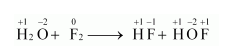# Fluorine reacts with ice and results in the change:

Question:

Fluorine reacts with ice and results in the change:

$\mathrm{H}_{2} \mathrm{O}(\mathrm{s})+\mathrm{F}_{2}(\mathrm{~g}) \rightarrow \mathrm{HF}(\mathrm{g})+\mathrm{HOF}(\mathrm{g})$

Justify that this reaction is a redox reaction.

Solution:

Let us write the oxidation number of each atom involved in the given reaction above its symbol as:Here, we have observed that the oxidation number of $\mathrm{F}$ increases from 0 in $\mathrm{F}_{2}$ to $+1$ in $\mathrm{HOF}$. Also, the oxidation number decreases from 0 in $\mathrm{F}_{2}$ to $-1$ in $\mathrm{HF}$. Thus, in the above reaction, $\mathrm{F}$ is both oxidized and reduced. Hence, the given reaction is a redox reaction.